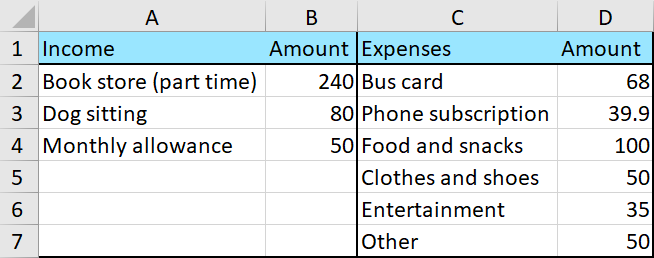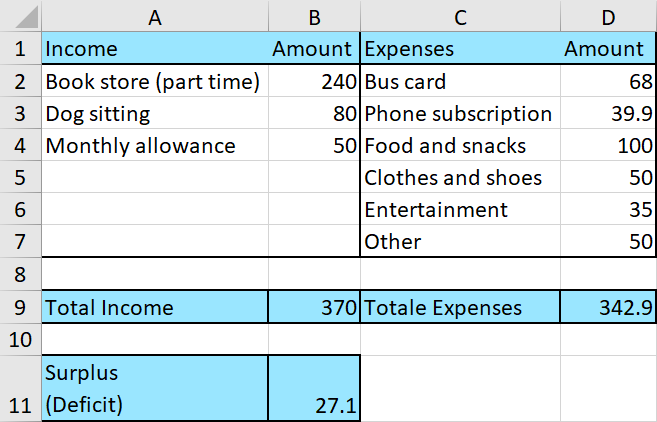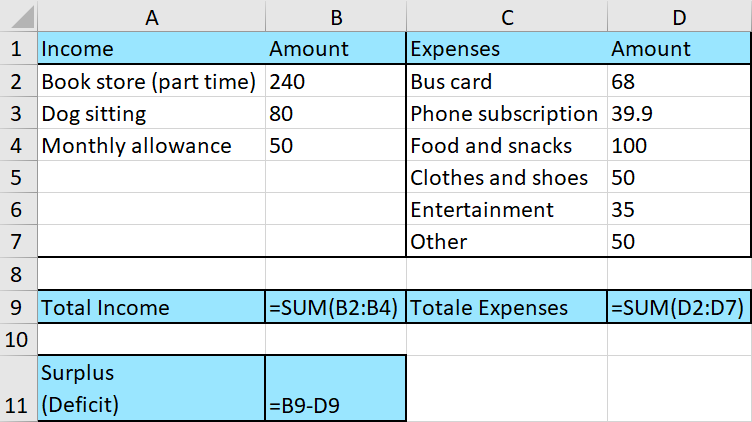Math Topics

# How to Create an Excel Budget

Here is a guide on how to make a budget in Excel. Making a budget is a useful aid to get a solid overview of your personal finances, or the finances of a company.

A budget consists mainly of the quite simple arithmetic operations addition and subtraction. This is something a spreadsheet can do for you with ease.

Excel Instruction

1.
Divide your different income and expenses into separate categories. These categories will depend on your sources of income (or the company’s). Do the same with your expenses.
2.
Enter the amounts for each category.
3.
4.
Lastly, calculate the projected surplus or deficit by subtracting expenses from income.

Example 1

Erica Clementine is making an overview of all the income and expenses she expects in a certain month. She needs your help to use Excel to create a budget.

1.
Below you have categorized Erica Clementine’s various income sources and expenses.

 Income Amount Expenses Amount Bookstore (part time) $$240$ Bus card$$68$ Dog sitting $$80$ Phone subscription$$39.90$ Monthly allowance $$50$ Food and snacks$$100$ Clothes and shoes $$50$ Entertainment$$35$ Other \$$50$

2.
Enter these numbers into Excel:3.
Eventually, sum all the income and expenses, and find Erica Clementine’s surplus/deficit:Here are the formulas used for each cell.Note! Notice that Erica Clementine’s budget had a surplus because the number in cell  B11 is positive. You should always set up budgets with a surplus.